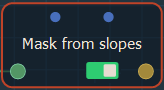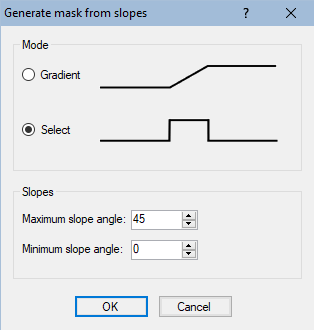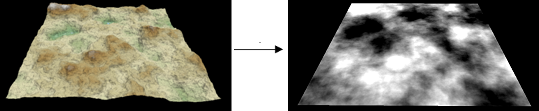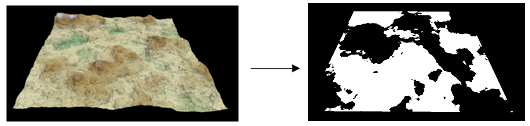This node creates a mask from the slopes of a terrain. The mask created has the same size and resolution as the input terrain.

To add a Mask from slopes node, right click in the Graph Editor and select Create Node  > Mask Generation  > Mask from slopes.Double click on the node to open its parameters:Editing a Mask from slopes node

Two modes exist to edit the Mask from slopes node.

• Gradient mode: In this mode, a gradient of values exists, with 0 corresponding to the defined Minimum slope angle and 1 corresponding to the defined Maximum slope angle.
At each vertex, the mask has a value of 0 (black color) if the slope of the terrain is less than or equal to the minimum slope angle; it has a value of 1 (white color) if the slope of the terrain is higher or equal to the maximum slope; it has a value between 0 and 1 if the slope of the terrain lies between these two slope.• Select mode: At each vertex, the mask has a value of 1 (white color) if the slope of the terrain is between the Maximum slope angle and the Minimum slope angle; otherwise, the mask is set to 0 (black color). The range is set manually.Parameters

Parameter Use
Gradient mode The mask takes a gradient with values between 0 for the minimum slope angle up to 1 for the maximum slope angle
Select mode The mask is set to 1 for slopes between minimum slope angle and maximum slope angle; otherwise it is set to 0
Maximum slope angle The maximum slope in degrees of the terrain
Minimum slope angle The minimum slope in degrees of the terrain